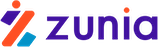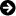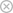Tuyển sinh 2023 dành cho Gen-Z# Tính tóan động đất 20

Chia sẻ: Tran Vu | Ngày: | Loại File: PDF | Số trang:16286
lượt xem
55

Tham khảo tài liệu 'tính tóan động đất 20', kỹ thuật - công nghệ, kiến trúc - xây dựng phục vụ nhu cầu học tập, nghiên cứu và làm việc hiệu quả

Chủ đề:

## Nội dung Text: Tính tóan động đất 20

1. Symbols Symbols A Cross-sectional area of a pile, Equation Aw Area of shear reinforcement, Equation (4-9) (7-12) Cross-sectional area of shear wall Nominal area of the web, Equation (5-7) boundary members or diaphragm chords Area of link stiffener web, Equation in.2, Equations (8-2), (8-4), (8-5) (5-28), (5-31) Area of the web cross section, = bwd, Ab Gross area of bolt or rivet, Equations Chapter 6 (5-18), (5-22), (5-24) Sum of net mortared area of bed joints Ax Accidental torsion amplification factor, above and below the test unit, Equation Equation (3-1) (7-2) B Width of footing, Equations (4-6), (4-7), Ac Area of column, Equation (5-8) (4-8) Ae Effective net area of the horizontal leg, B1 Damping coefficient used to adjust one- Equation (5-20) second period spectral response for the effect of viscous damping, , Equations Ag Gross area of the horizontal leg, Equation (1-10), (1-11) (5-19) Gross area of cast iron column, Equation BD1 Numerical damping coefficient taken (5-36) equal to the value of B1, as set forth in Gross area of column, in.2, Equation (6-4) Table 1-6, at effective damping β equal to the value of βD, Equation (9-2) Aj Effective cross-sectional area of a beam- column joint, in.2, in a plane parallel to BM1 Numerical damping coefficient taken plane of reinforcement generating shear in equal to the value of B1, as set forth in the joint calculated as specified in Table 1-6, at effective damping β equal to Section 6.5.2.3.1, Equation (6-5) the value of βM, Equation (9-4) An Area of net mortared/grouted section, BS Coefficient used to adjust short-period Equations (7-1), (7-3), (7-5), (7-7), (7-9), spectral response for the effect of viscous (7-10), (7-11), (7-13) damping, Equations (1-8), (1-9), (1-11) Ani Area of net mortared/grouted section of C (or Cj ) Damping coefficient for viscoelastic masonry infill, Equation (7-15) device (or device j), Equations (9-22), (9-24), (9-29), (9-30), (9-35), (9-37) As Area of nonprestressed tension reinforce- ment, in.2, Tables 6-18, 6-20 C0 Modification factor to relate spectral Area of reinforcement, Equation (7-13) displacement of an equivalent SDOF sys- tem to the roof displacement of the build- A′s Area of compression reinforcement, in.2, ing MDOF system, Equation (3-15) Tables 6-18, 6-20 Damping coefficient for fluid-viscous device, Equation (9-25) C1 Modification factor to relate expected maximum inelastic displacements to dis- placements calculated for linear elastic response, Equations (3-5), (3-6), (3-10), (3-15), (3-19) FEMA 356 Seismic Rehabilitation Prestandard Symbols-1
2. Symbols C2 Modification factor to represent the effects DCR Demand-capacity ratio, computed in of pinched hysteresis shape, stiffness deg- accordance with Equation (2-1) or required radation and strength deterioration on the in Equation (2-2) maximum displacement response, ____ Average demand-capacity ratio for a story, Equations (3-5), (3-6), (3-10), (3-15), DCR (3-19) computed in accordance with Equation (2-2) C3 Modification factor to represent increased displacements due to p-∆ effects, Equa- DD Design displacement, in. (mm) at the cen- tions (3-5), (3-6), (3-10), (3-15), (3-17), ter of rigidity of the isolation system in the (3-19) direction under consideration, Equations (9-2), (9-6), (9-8), (9-10), (9-14), (9-15), Cb Coefficient to account for effect of nonuni- (9-18), (9-22) form moment given in AISC (1993) LRFD Specifications, Equation (5-9) D′D Design Earthquake target displacement, in. (mm) at a control node located at the cen- CFi Stage combination factors for use with ter of mass of the first floor above the velocity-dependent energy dissipation isolation system in the direction under con- devices as calculated by Equations (9-31) sideration, as prescribed by Equation or (9-32) (9-10) Cm Effective mass factor from Table 3-1, DM Maximum displacement, in. (mm) at the Equations (3-10), (3-16) center of rigidity of the isolation system in the direction under consideration, Ct Numerical value for adjustment of period Equations (9-4), (9-7), (9-11), (9-16), T, Equation (3-7) (9-17), (9-19) Cvx Vertical distribution factor for the pseudo D′M BSE-2 target displacement, in. (mm) at a lateral load, Equations (3-11), (3-12) control node located at the center of mass D Generalized deformation, unitless of the first floor above the isolation system in the direction under consideration, as Relative displacement between two ends prescribed by Equation (9-11) of an energy dissipation unit, Equations (9-1), (9-20), (9-22) Dp Relative seismic displacement that the component must be designed to accommo- D– Maximum negative displacement of an date, Equations (11-8), (11-9), (11-10), energy dissipation unit, Equations (9-21), (11-11) (9-23) Dr Drift ratio for nonstructural components, D+ Maximum positive displacement of an Equation (11-7) energy dissipation unit, Equations (9-21), (9-23) DTD Total design displacement, in. (mm) of an • element of the isolation system, including D Relative velocity between two ends of an both translational displacement at the energy dissipation unit, Equations (9-22), center of rigidity and the component of (9-25) torsional displacement in the direction Dave Average displacement of an energy dissi- under consideration, as specified by pation unit, equal to (|D+| + |D–|)/2, Equation (9-6) Equation (9-24) Dclear Required clearance between a glass component and the frame, Equation (11-9) Symbols-2 Seismic Rehabilitation Prestandard FEMA 356
3. Symbols DTM Total maximum displacement, in. (mm) of Fcr Allowable axial buckling stress, see an element of the isolation system, Equation (5-36) including both translational displacement at the center of rigidity and the component FEXX Classification strength of weld metal, of torsional displacement in the direction Chapter 5 under consideration, as specified by Fi Inertia force at floor level i, Equation Equation (9-7) (9-27) E Young’s modulus of elasticity, Equations Lateral load applied at floor level i, (4-9), (5-1), (5-2), (5-17), (8-2), (8-4), Equation (3-13) (8-5) Fmi m-th mode horizontal inertia force at floor Ec Modulus of elasticity of concrete, psi, level i, Equation (9-34) Equation (6-6) Fp Horizontal seismic force for design of a Efe Expected elastic modulus of frame structural or nonstructural component and material, ksi, Equation (7-14) its connection to the structure, Equations (2-3), (2-4), (2-5), (2-6), (2-7) ELoop Energy dissipated, in kip-inches (kN-mm), Component seismic design force applied in an isolator unit during a full cycle of horizontally at the center of gravity of the reversible load over a test displacement component or distributed according to the range from ∆+ to ∆-, as measured by the mass distribution of the component, area enclosed by the loop of the Equations (11-1), (11-2), (11-3), (11-4) force-deflection curve, Equation (9-13) Fpv Component seismic design force applied Eme Expected elastic modulus of masonry in vertically at the center of gravity of the compression as determined per component or distributed according to the Section 7.3.2.4, Equation (7-14) mass distribution of the component, Equations (11-2), (11-5), (11-6) Es Modulus of elasticity of reinforcement, psi, Chapter 6 Fpx Diaphragm lateral force at floor level x, Equation (3-13) Ese Expected elastic modulus of reinforcing steel per Section 7.3.2.8 Fte Expected tensile strength, Equations (5-20), (5-22), (5-24) F Force in an energy dissipation unit, Equations (9-1), (9-20), (9-22), (9-25) Fv Factor to adjust spectral acceleration at one second for site class, Equation (1-8) F– Negative force, in k, in an isolator or Design shear strength of bolts or rivets, energy dissipation unit during a single Chapter 5 cycle of prototype testing at a displace- ment amplitude of ∆−, Equations (9-12), Fve Unfactored nominal shear strength of bolts (9-21), (9-23), (9-38) or rivets given in AISC(1993) LRFD Specifications, Equation (5-18) F+ Positive force, in k, in an isolator or energy Fx Lateral load applied at floor level x, dissipation unit during a single cycle of prototype testing at a displacement Equation (3-11) amplitude of ∆+, Equations (9-12), (9-21), Fy Specified minimum yield stress for the (9-23), (9-38) type of steel being used, Equation (5-7) Fa Factor to adjust spectral acceleration in the Fyb Fy of a beam, Chapter 5 short-period range for site class, Equation (1-7) Fyc Fy of a column, Chapter 5 FEMA 356 Seismic Rehabilitation Prestandard Symbols-3
4. Symbols Fye Expected yield strength, Equations (5-1) to J A coefficient used in linear procedures to (5-8), (5-19), (5-23), (5-25), (5-31), (5-34) estimate the actual forces delivered to force-controlled components by other Fyf Fy of a flange, Chapter 5 (yielding) components, Equations (3-5), (3-19) FyLB Lower-bound yield strength, Chapter 5 K Length factor for brace; defined in AISC G Soil Shear modulus, Equation (4-6) (1993) LRFD Specifications, Chapter 5 Shear modulus of steel, Equations (5-28), (5-33) K’ Storage stiffness as prescribed by Equation Modulus of rigidity of wood structural (9-23) panels, psi, Equations (8-2), (8-4), (8-5) K" Loss stiffness as prescribed by Equation Gd Shear stiffness of shear wall or diaphragm (9-24) assembly, Equations (8-1), (8-3) Kθ Rotational stiffness of a partially restrained Gme Shear modulus of masonry as determined connection, Equations (5-15), (5-16), per Section 7.3.2.7 (5-17) Go Initial or maximum shear modulus, Kb Flexural stiffness, Equations (5-27), (5-29) Equations (4-4), (4-5) KDmax Maximum effective stiffness, in k/in., of H Thickness of a soil layer in feet, the isolation system at the design displace- Chapter 11 ment in the horizontal direction under Horizontal load on footing, Chapter 4 consideration, as prescribed by Equation (9-14) Hrw Height of the retaining wall, Equation (4-11) KDmin Minimum effective stiffness, in k/in. (kN/ mm), of the isolation system at the design I Moment of inertia, Equation (6-6) displacement in the horizontal direction Ib Moment of inertia of a beam, Equations under consideration, as prescribed by (5-1), (5-17) Equation (9-15) Ic Moment of inertia of a column, Equation Ke Effective stiffness of the building in the (5-2) direction under consideration, for use with the NSP, Equation (3-14) Icol Moment of inertia of column section, Elastic stiffness of a link beam, Equation (7-14) Equations (5-27), (5-30) If Moment of inertia of most flexible frame Ki Elastic stiffness of the building in the member confining infill panel, Chapter 7 direction under consideration, for use with the NSP, Equation (3-14) Ig Moment of inertia of gross concrete sec- tion about centroidal axis, neglecting KMmax Maximum effective stiffness, in k/in., of reinforcement, Chapter 6 the isolation system at the maximum displacement in the horizontal direction Ip Component performance factor; 1.0 shall under consideration, as prescribed by be used for the Life Safety Nonstructural Equation (9-16) Performance Level and 1.5 shall be used for the Immediate Occupancy Nonstruc- KMmin Minimum effective stiffness, in k/in., of tural Performance Level, Equations (11-1), the isolation system at the maximum (11-3), (11-4), (11-5), (11-6) displacement in the horizontal direction under consideration, as prescribed by Equation (9-17) Symbols-4 Seismic Rehabilitation Prestandard FEMA 356
7. Symbols QG Design action due to gravity loads, SXS Spectral response acceleration parameter Equation (3-3), (3-4), (3-18), (3-19) at short periods for the selected Earthquake Hazard Level and damping, adjusted for QL Design action due to live load, Equations site class, and determined in accordance (3-3), (3-4) with Section 1.6.1.4 or 1.6.2.1, QS Design action due to snow load, Equations Equation (1-4), (1-8), (1-9), (1-11), (1-13), (3-3), (3-4) (1-14), (1-15), (1-16), (4-11), (11-1), (11-3), (11-4), (11-5), (11-6) QUD Deformation-controlled design action due to gravity and earthquake loads, T Fundamental period of the building in the Equations (2-1), (3-18), (3-20) direction under consideration, seconds, Equations (1-8), (1-10), (3-7), (3-8), QUF Force-controlled design action due to grav- (3-9), (3-10), (9-29) ity and earthquake loads, Equations (3-19), Tensile load in column, Equation (5-13) (3-21)) T0 Period at which the constant acceleration Qy Yield strength of a component, region of the design response spectrum Figures 2-3, 2-5 begins at a value = 0.2TS, Equations (1-8), Substitute yield strength, Figure 2-5 (1-12) Q′y TCE Expected tensile strength of column com- R Ratio of the elastic-strength demand to the puted in accordance with Equation (5-8) yield-strength coefficient, Equations (3-15), (3-16), (3-17) TD Effective period, in seconds, of the seismic-isolated structure at the design ROT Response modification factor for overturn- displacement in the direction under ing moment MOT, Equation (3-6) consideration, as prescribed by Equation (9-3) Rp Component response modification factor from Table 11-2, Equation (11-3)) Te Effective fundamental period of the building in the direction under consider- S1 Spectral response acceleration parameter ation, for use with the NSP, Equations at a one-second period, obtained from (3-14), (3-15), (3-17) response acceleration maps, Equations Effective fundamental period, in seconds, (1-1), (1-3), (1-5) of the building structure above the Sa Spectral response acceleration, g, isolation interface on a fixed base in the Equations (1-8), (1-9), (1-10), (3-10), direction under consideration, Equations (3-15), (3-16) (9-10), (9-11) Sn Distance between nth pile and axis of rota- Ti Elastic fundamental period of the building tion of a pile group, Equation (4-10) in the direction under consideration, for use with the NSP, Equation (3-14) SS Spectral response acceleration parameter at short periods, obtained from response TM Effective period, in seconds, of the seis- acceleration maps, Equations (1-1), mic-isolated structure at the maximum dis- (1-3), (1-7) placement in the direction under consideration, as prescribed by Equation SX1 Spectral response acceleration parameter (9-5) at a one-second period for any earthquake hazard level and any damping, adjusted for Tm m-th mode period of the rehabilitated site class, Equations (1-5), (1-10), (1-11), building including the stiffness of the (1-13), (1-14), (1-15), (1-16) velocity-dependent devices, Equation (9-35) FEMA 356 Seismic Rehabilitation Prestandard Symbols-7
8. Symbols TS Period at which the constant acceleration Vi The total calculated lateral shear force in region of the design response spectrum the direction under consideration in an transitions to the constant velocity region, element or at story i due to earthquake Equations (1-8), (1-9), (1-10), (1-11), response to the selected ground shaking (1-12), (1-13), (3-10), (3-15) level, as indicated by the selected linear analysis procedure, Equations (2-2), (3-2) Tss Secant fundamental period of a rehabili- tated building calculated using Equation Vine Expected shear strength of infill panel, (3-14) but replacing the effective stiffness Equation (7-15) (Ke) with the secant stiffness (Ks) at the VmL Lower bound shear strength provided by target displacement, Equation (9-37) masonry, Equations (7-8), (7-11) V Pseudo lateral load, Equations (3-10), Vn Nominal shear strength at section, (3-11) Equation (6-5) Design shear force at section, Equation (6-4) Vo Shear strength of slab at critical section, Shear on masonry section, Equation (7-11) Chapter 6 V* Modified equivalent base shear, Chapter 9 Vpz Panel zone shear, Chapter 5 Vb The total lateral seismic design force or Vr Expected shear strength of wall or pier shear on elements of the isolation system based on rocking shear, Equation (7-4) or elements below the isolation system, as prescribed by Equation (9-8) Vs Nominal shear strength provided by shear reinforcement, Chapter 6 Vbjs Expected shear strength of wall or pier The total lateral seismic design force or based on bed-joint sliding shear stress, see shear on elements above the isolation Equation (7-3) system, as prescribed by Section 9.2.4.4.2, Equation (9-9) Vc Nominal shear strength provided by concrete, Equation (6-4) VsL Lower bound shear strength provided by shear reinforcement, Equations (7-8), VCE Expected shear strength of a member, (7-12) Equations (5-11), (5-31), (5-32), (5-34) Vt Base shear in the building at the target displacement, Chapter 3 VCL Lower bound shear strength, Equations (7-8), (7-9), (7-10) Vtc Lower bound shear strength based on toe compressive stress for wall or pier, Vdt Lower bound shear strength based on Chapter 7 diagonal tension stress for wall or pier, Chapter 7 Vtest Test load at first movement of a masonry unit, Equation (7-2) Vfre Expected story shear strength of the bare steel frame taken as the shear cpacity of Vu Factored shear force at section, Chapter 6 the column, Chapter 7 Vy Yield strength of the building in the direc- Vg Shear acting on slab critical section due to tion under consideration, for use with the gravity loads, Chapter 6 NSP, Equation (3-16) Vya Nominal shear strength of a member modi- fied by the axial load magnitude, Chapter 5 Symbols-8 Seismic Rehabilitation Prestandard FEMA 356